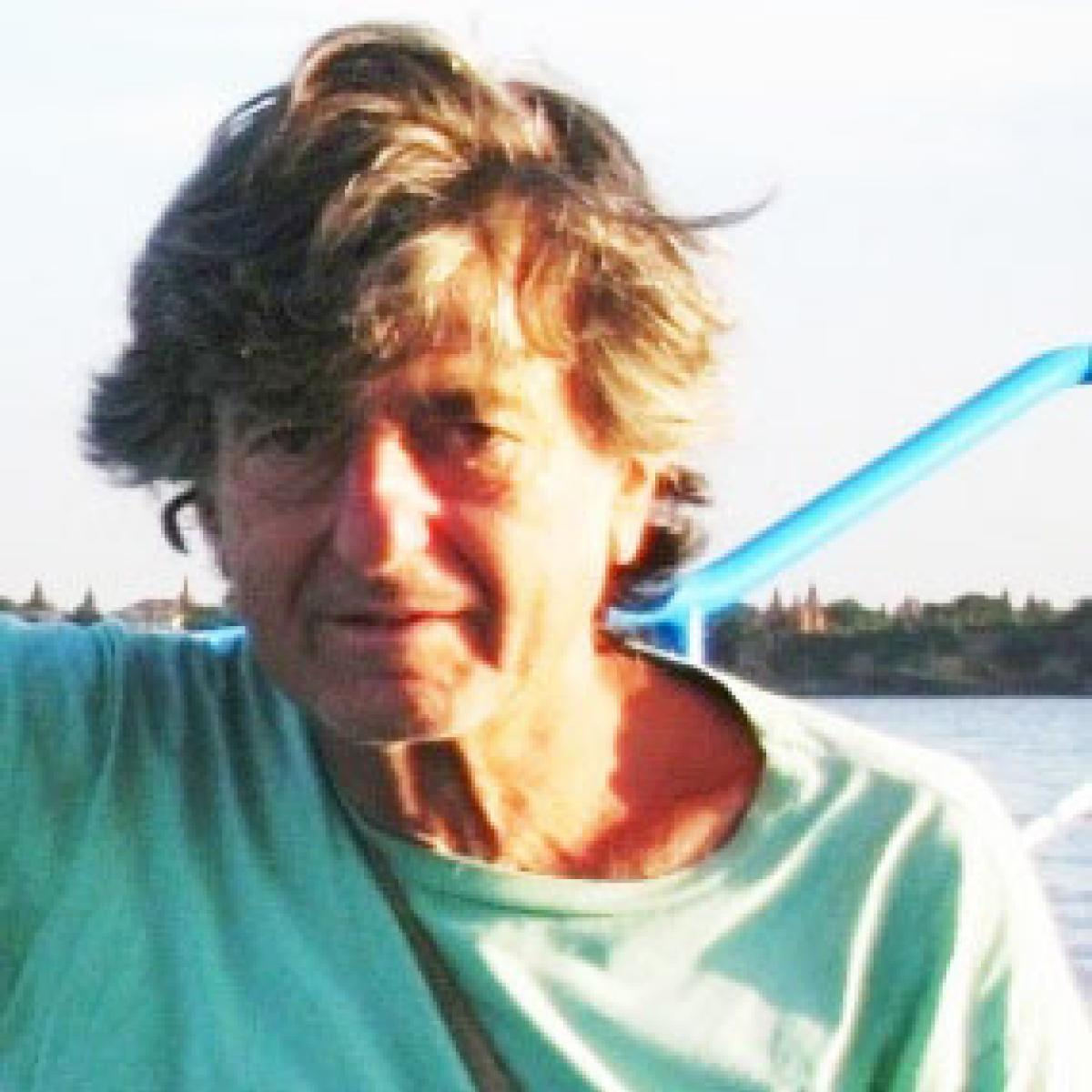# Michael Shub

Professor, City University of New York

Michael Shub began his mathematical career as a dynamicist. He defined and laid the groundwork for the classification of expanding maps which was ultimately completed by Gromov. These maps provided the first examples of structurally stable strange attractors, thus contributing in an essential way to the revolution in dynamical systems theory now known as chaos theory. He studied the relations between smooth dynamics and algebraic topology formulating the entropy conjecture. The conjecture was ultimately proven by Yomdin. With Charles Pugh he has worked on the extent that chaotic dynamical systems may be understood statistically. Their conjectures have been verified for a large set of cases. With Steve Smale he began working on the complexity theory of solving first one and then a system of polynomial equations. With Lenore Blum they generalized the theory of complexity of computation to the reals or complex numbers thus generalizing such problems as "Does P=NP?"